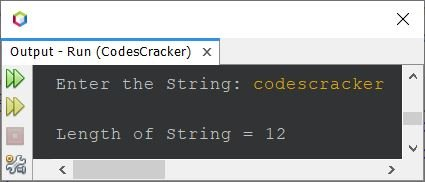# Java Program to Find Length of a String

This article covers multiple programs in Java that find and prints the length of a string entered by user at run-time of the program. Here are the list of programs covered by this article:

• Find length of a string without using length() method
• Find length of a string without using length() and toCharArray() methods
• Find length of string using length() method

## Find Length of String without using length() Method

The question is, write a Java program to find the length of a given string. Answer to this question, is the program given below:

```import java.util.Scanner;

public class CodesCracker
{
public static void main(String[] args)
{
String str;
int len=0;
Scanner scan = new Scanner(System.in);

System.out.print("Enter the String: ");
str = scan.nextLine();

char[] strChars = str.toCharArray();
for(char ch: strChars)
len++;

System.out.println("\nLength of String = " +len);
}
}```

The snapshot given below shows the sample run of above program with user input codescracker as string to find and print its length:## Find Length of String without length() and toCharArray()

This program is created using try...catch block. That is, the string gets fetched character by character. The fetching code, is enclosed within a try block, so that, while fetching after the last character, an exception gets caught. And using the catch block, I've printed the value of i, that equals the length of string.

```import java.util.Scanner;

public class CodesCracker
{
public static void main(String[] args)
{
int i=0;
Scanner scan = new Scanner(System.in);

System.out.print("Enter the String: ");
String str = scan.nextLine();

while(true)
{
try
{
char ch = str.charAt(i);
i++;
}
catch(Exception e)
{
System.out.println("\nLength of String = " +i);
break;
}
}
}
}```

## Find Length of String using length()

Following is the last program of this article, created using a library function, length() to do the job of finding the length of a string.

```import java.util.Scanner;

public class CodesCracker
{
public static void main(String[] args)
{
Scanner scan = new Scanner(System.in);

System.out.print("Enter the String: ");
String str = scan.nextLine();

int len = str.length();
System.out.println("\nLength of String = " +len);
}
}```

Also the following two statements, from above program:

```int len = str.length();
System.out.println("\nLength of String = " +len);```

can be replaced directly using single statement, as given below:

`System.out.println("\nLength of String = " +str.length());`

#### Same Program in Other Languages

Java Online Test

« Previous Program Next Program »